# RD Sharma Solutions for Class 10 Maths Chapter 3 Pair of Linear Equations In Two Variables Exercise 3.3

Apart from the graphical methods of solving simultaneous linear equations in two variables, we also have the algebraic methods too. Under the algebraic methods of solving simultaneous linear equations in two variables, we have three methods. Method of elimination by substitution and method of elimination by equating the coefficients are the prominent concepts covered in this exercise. Students can make use of the RD Sharma Solutions Class 10 Maths Chapter 3 Pair of Linear Equations In Two Variables Exercise 3.3 PDF given below for any clarifications related to these exercise problems.

## RD Sharma Solutions for Class 10 Maths Chapter 3 Pair of Linear Equations In Two Variables Exercise 3.3 Download PDF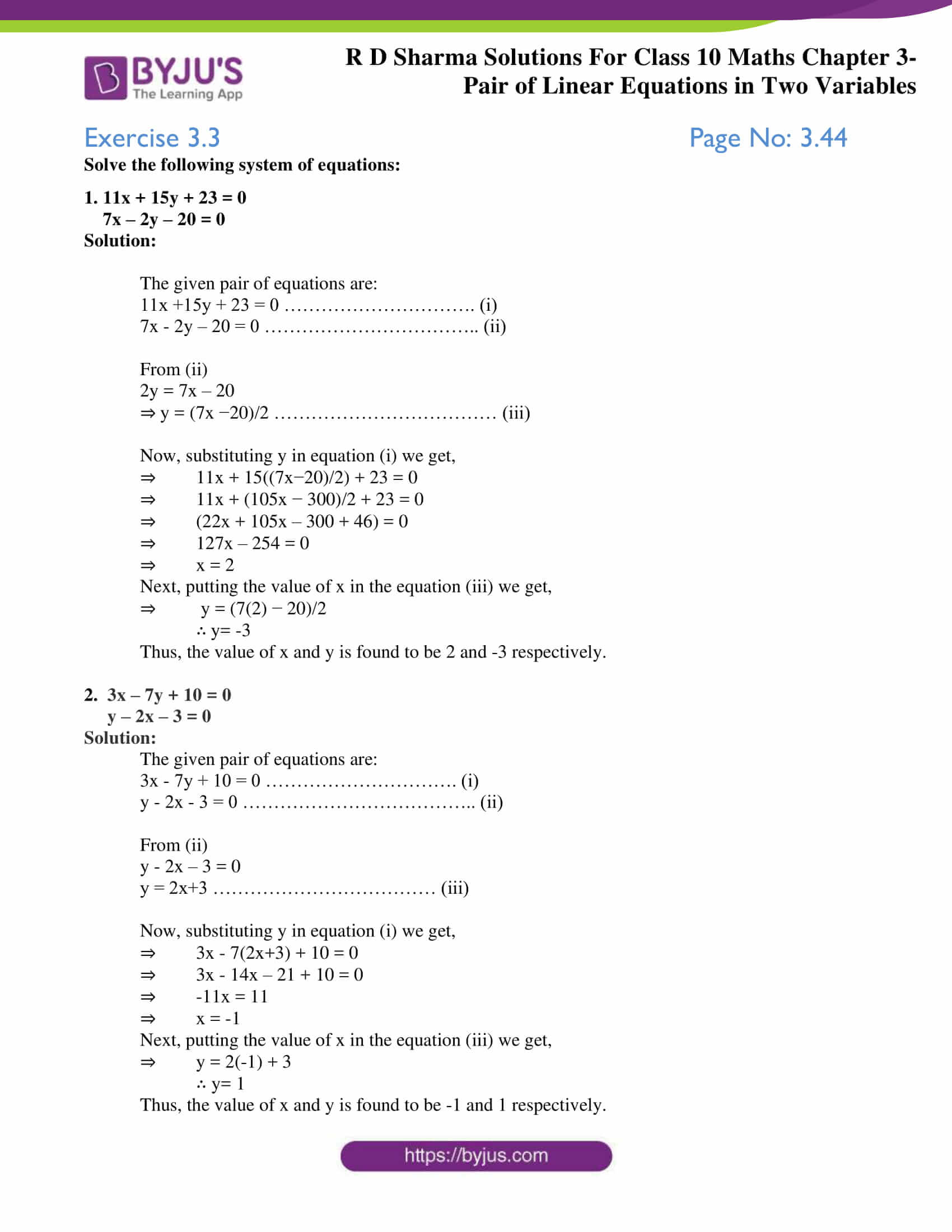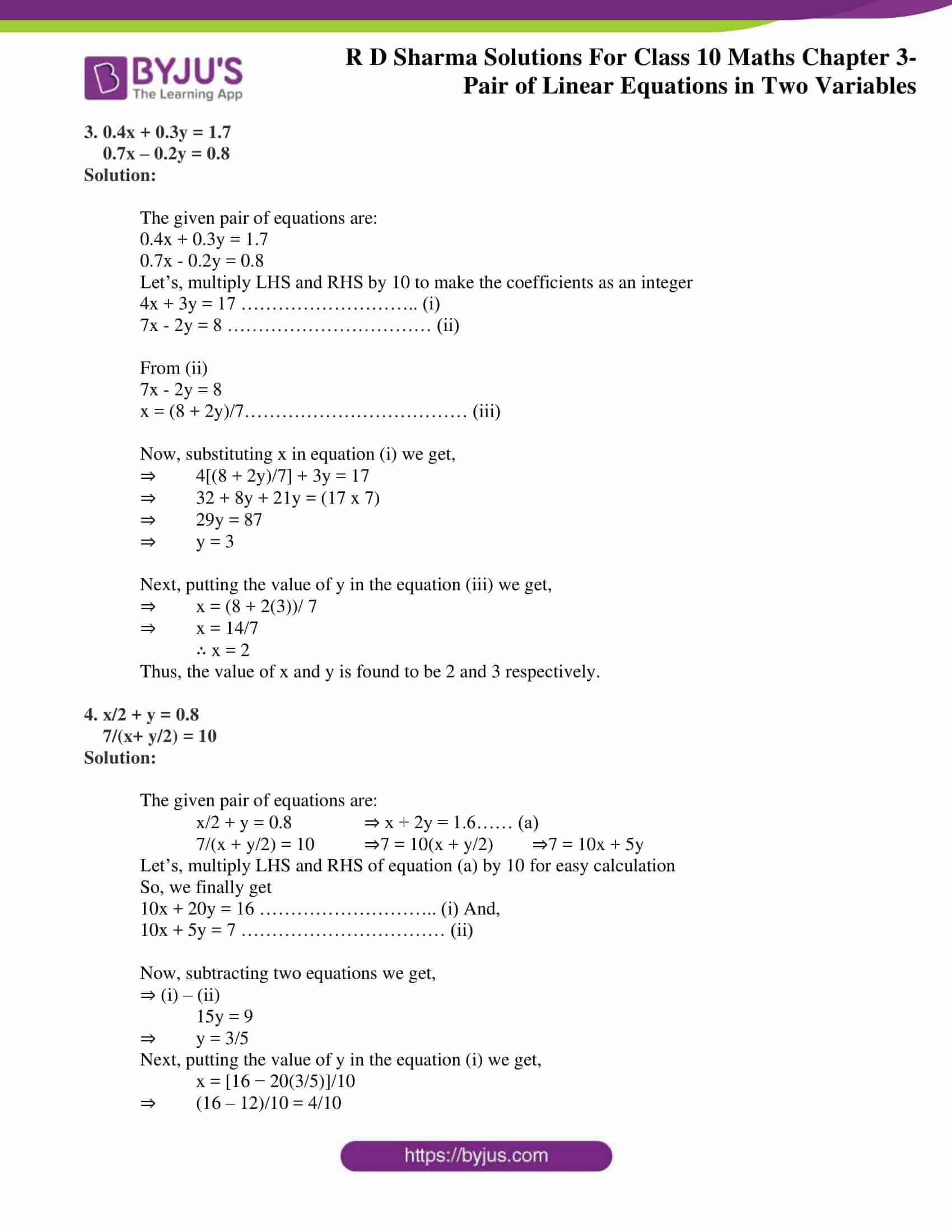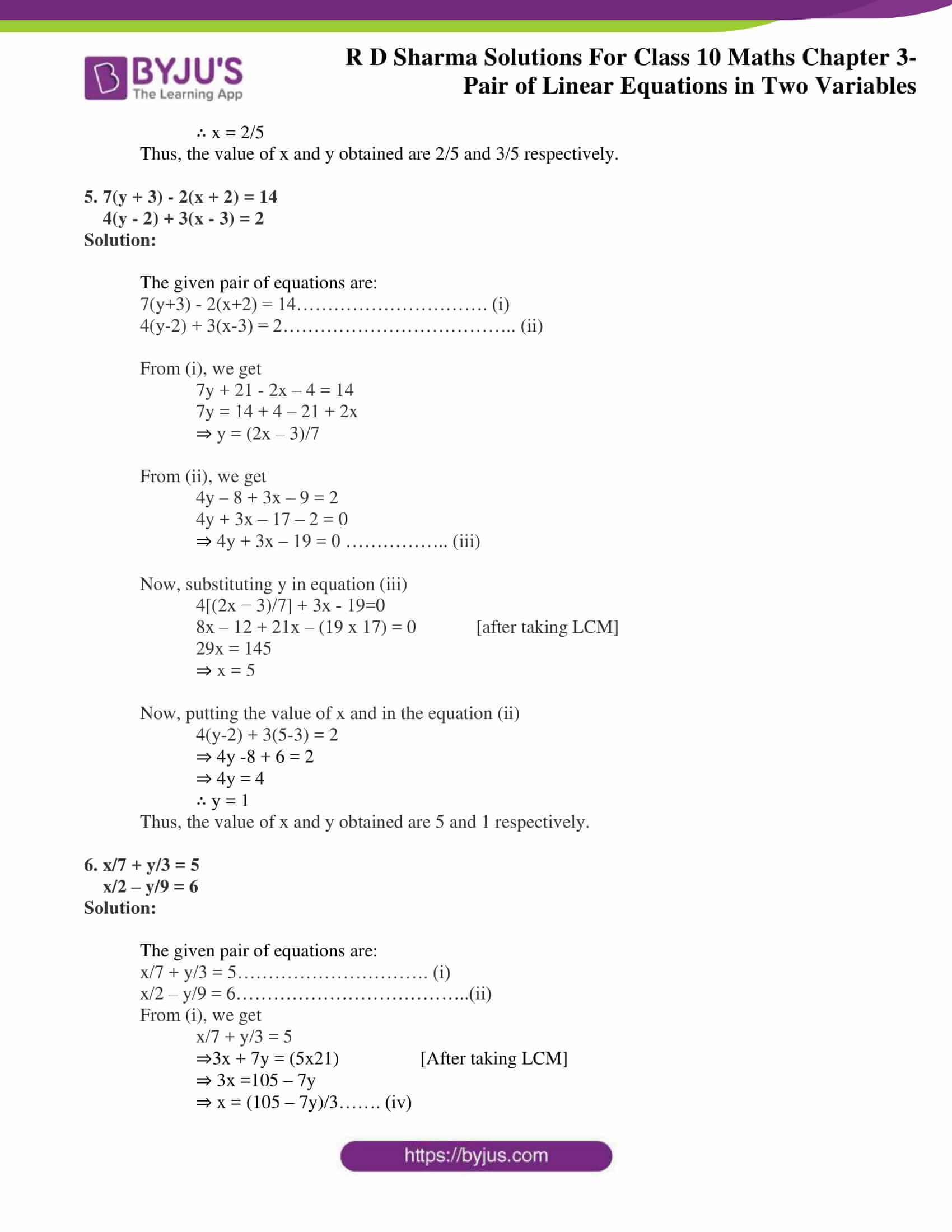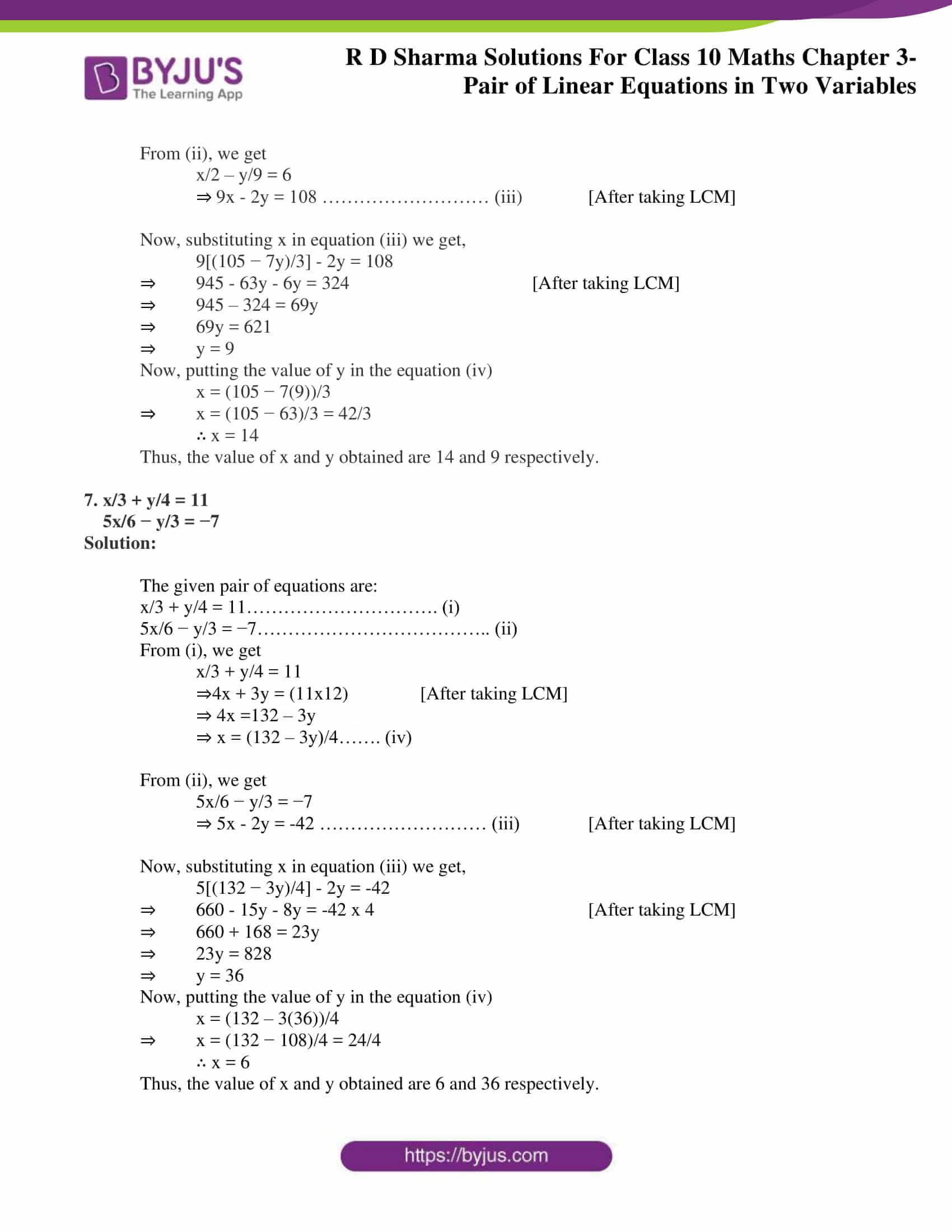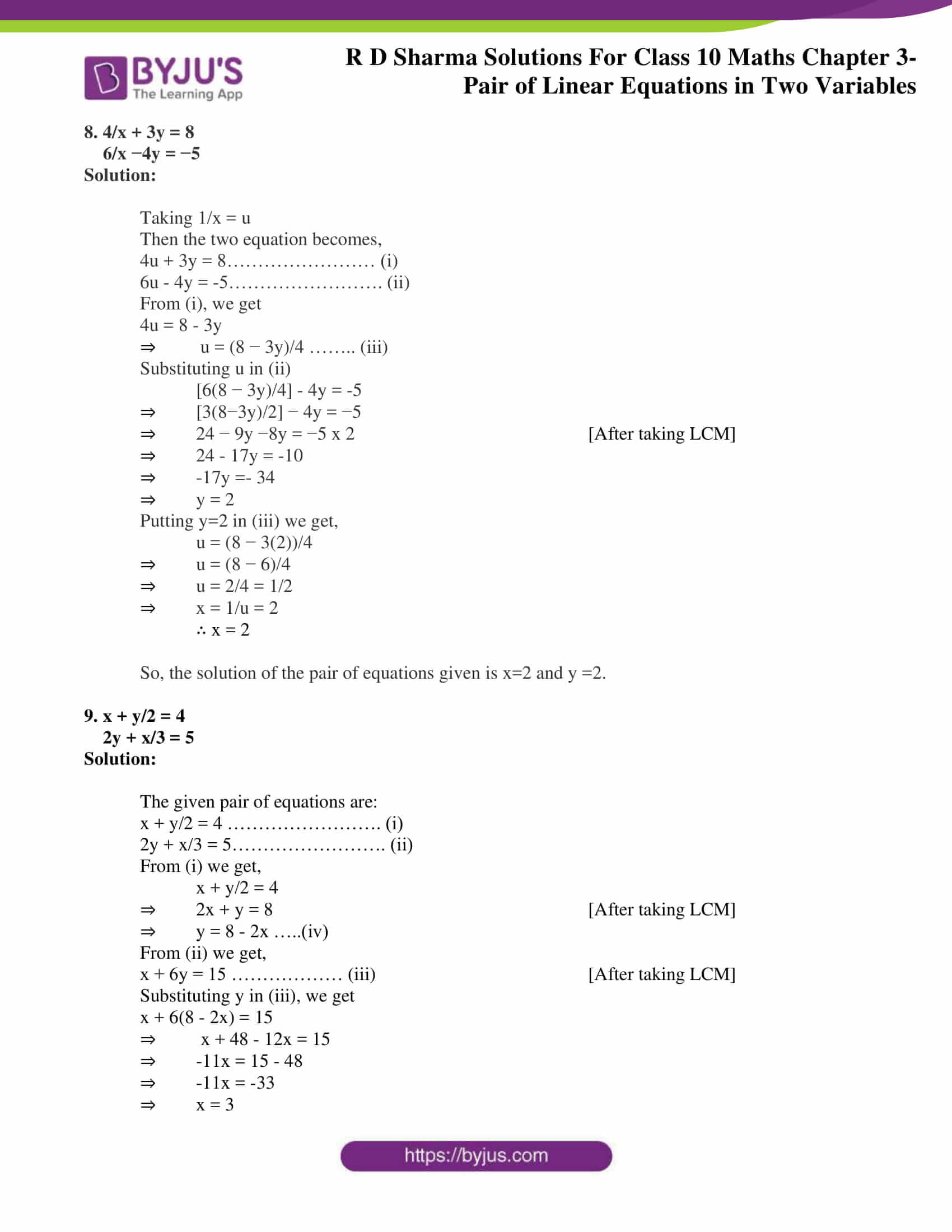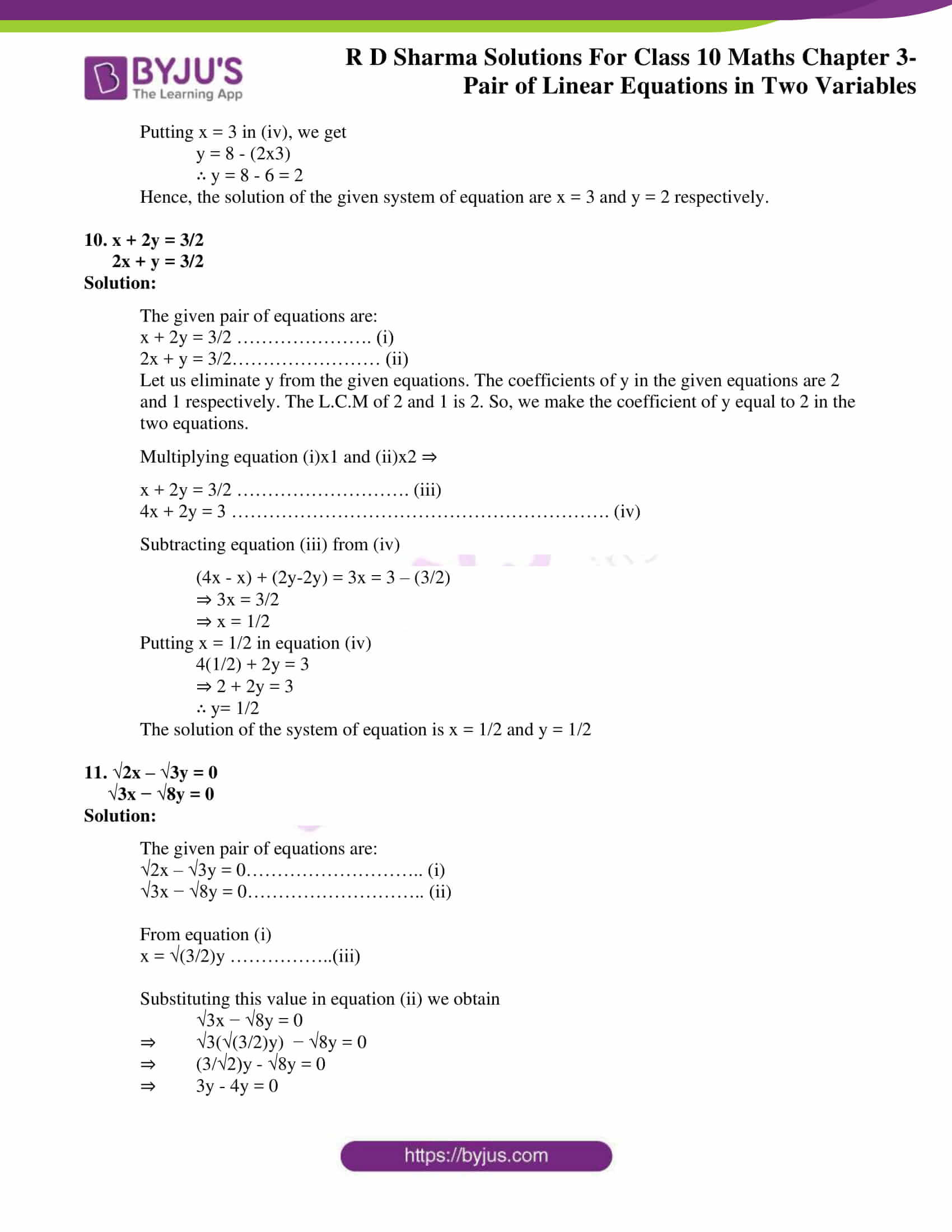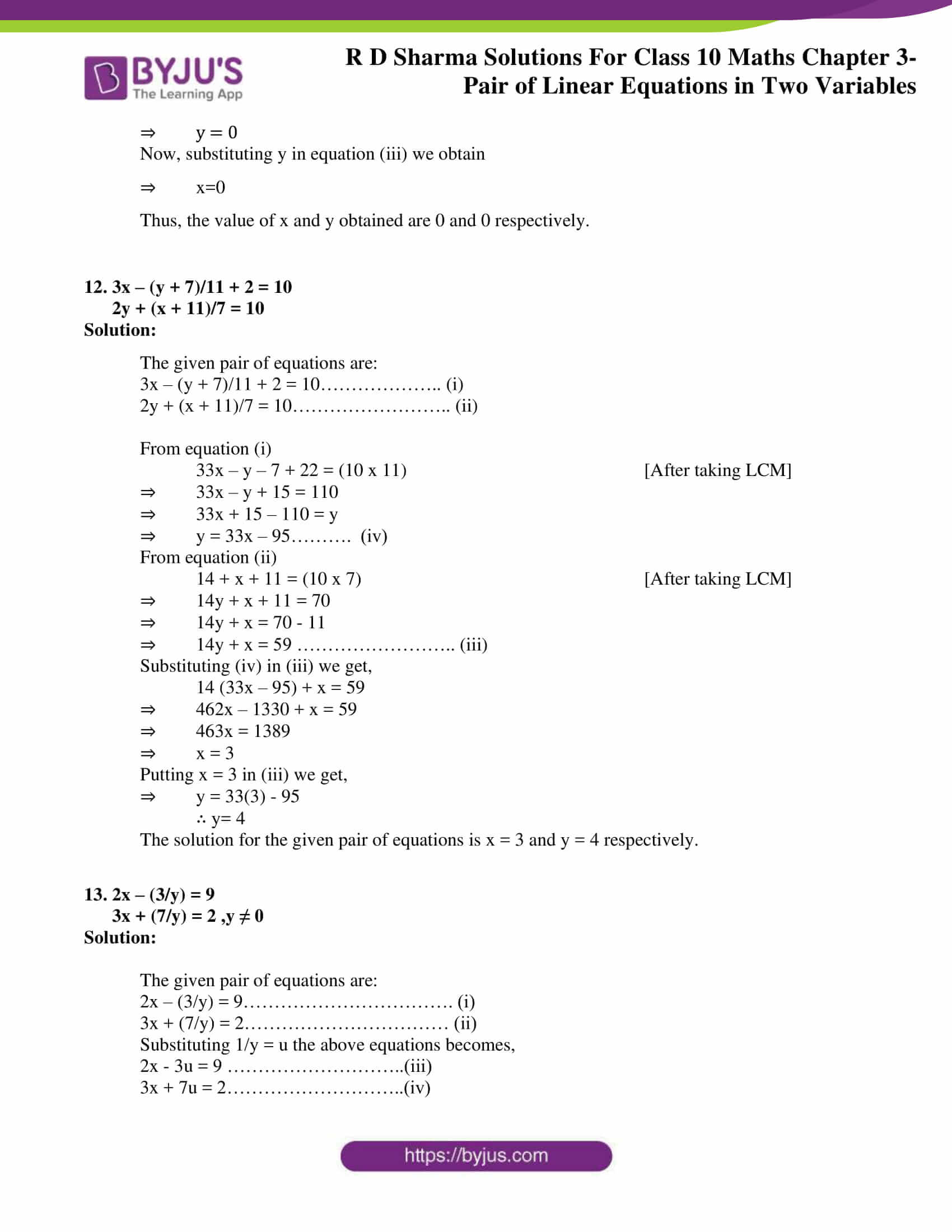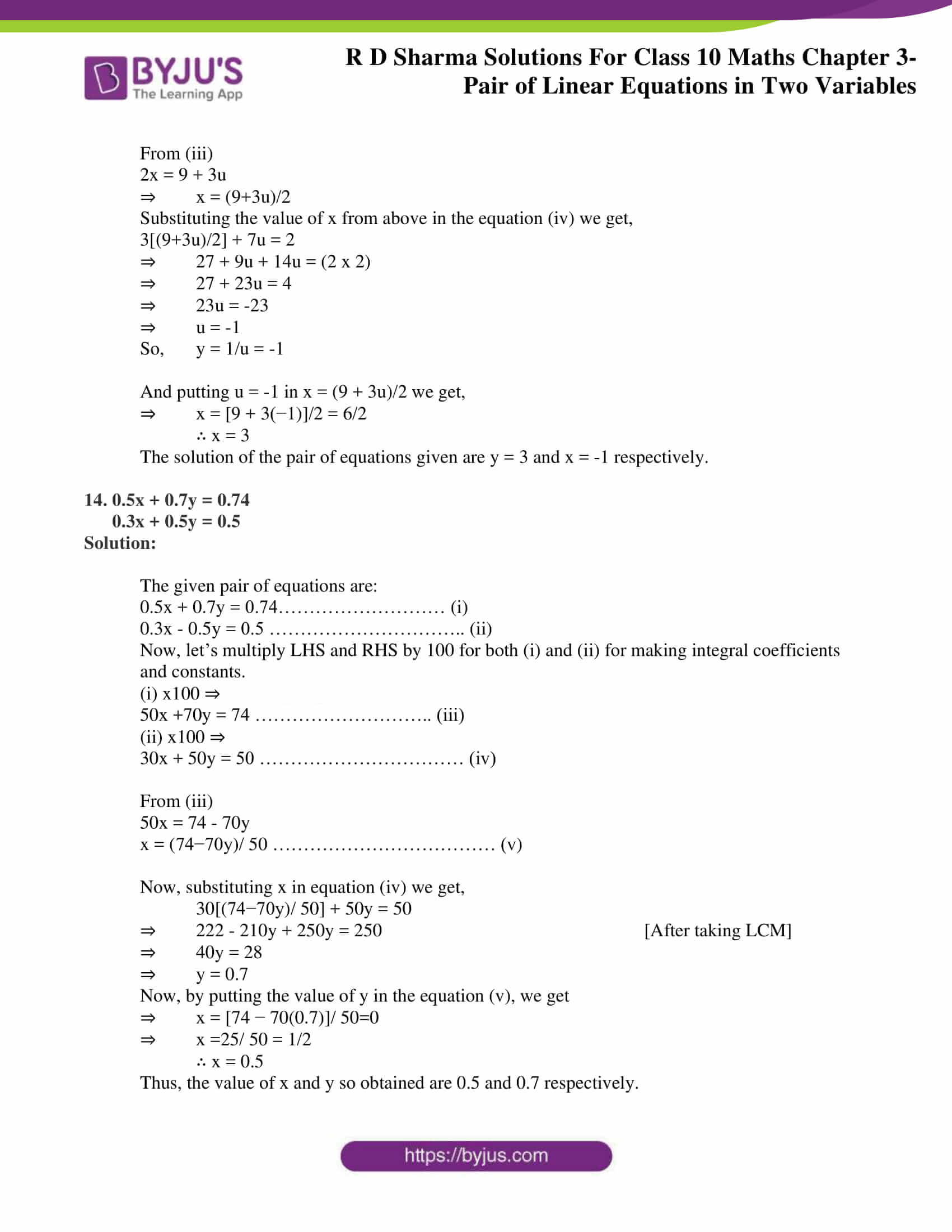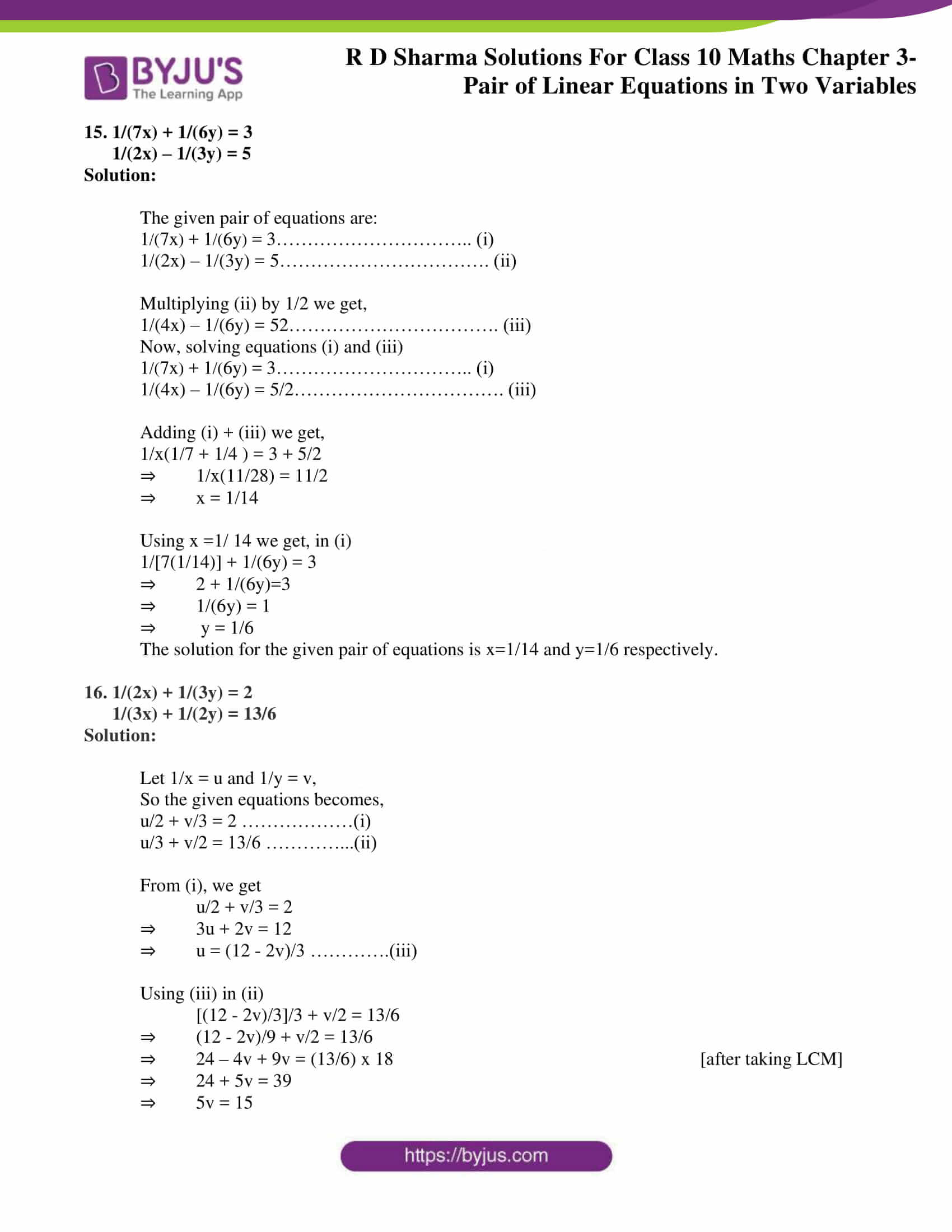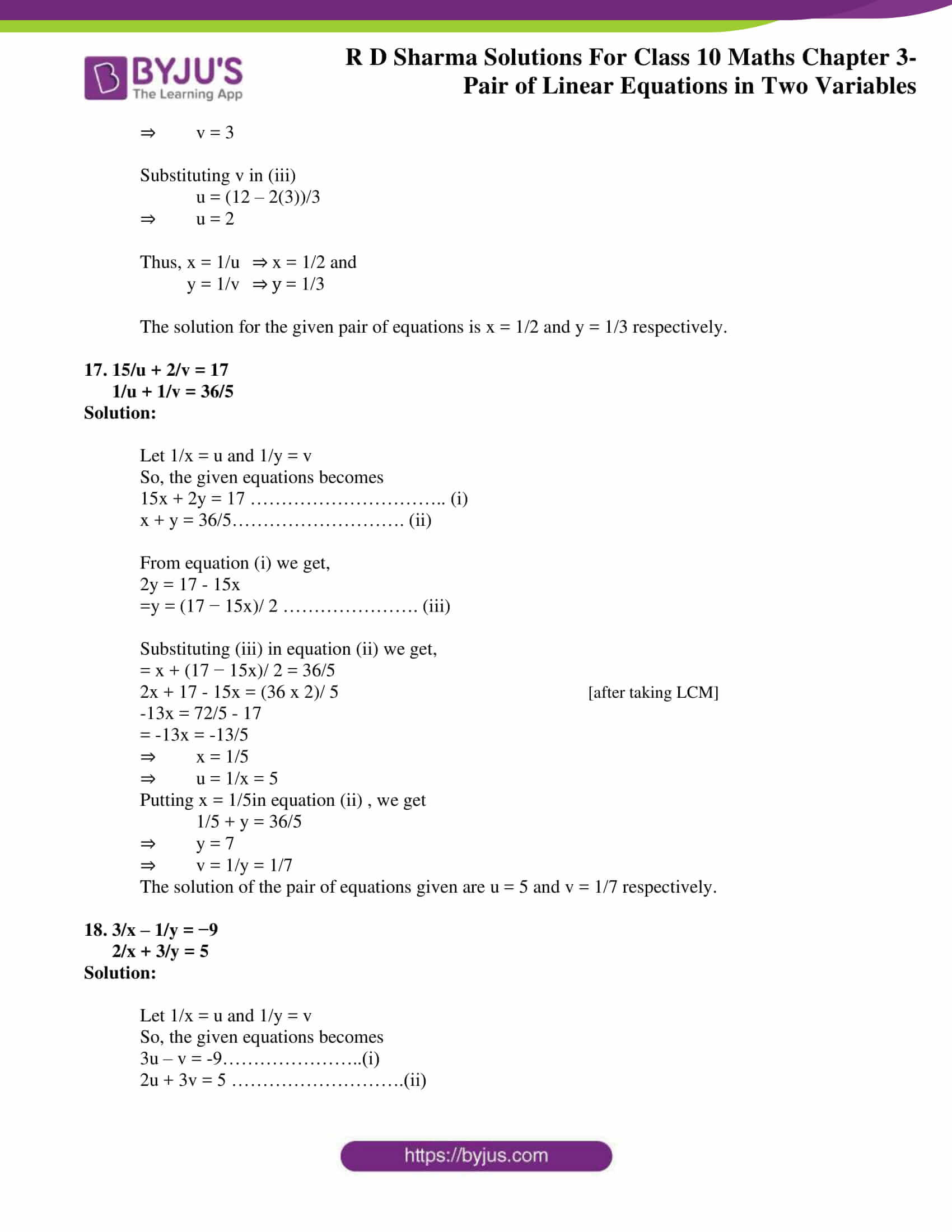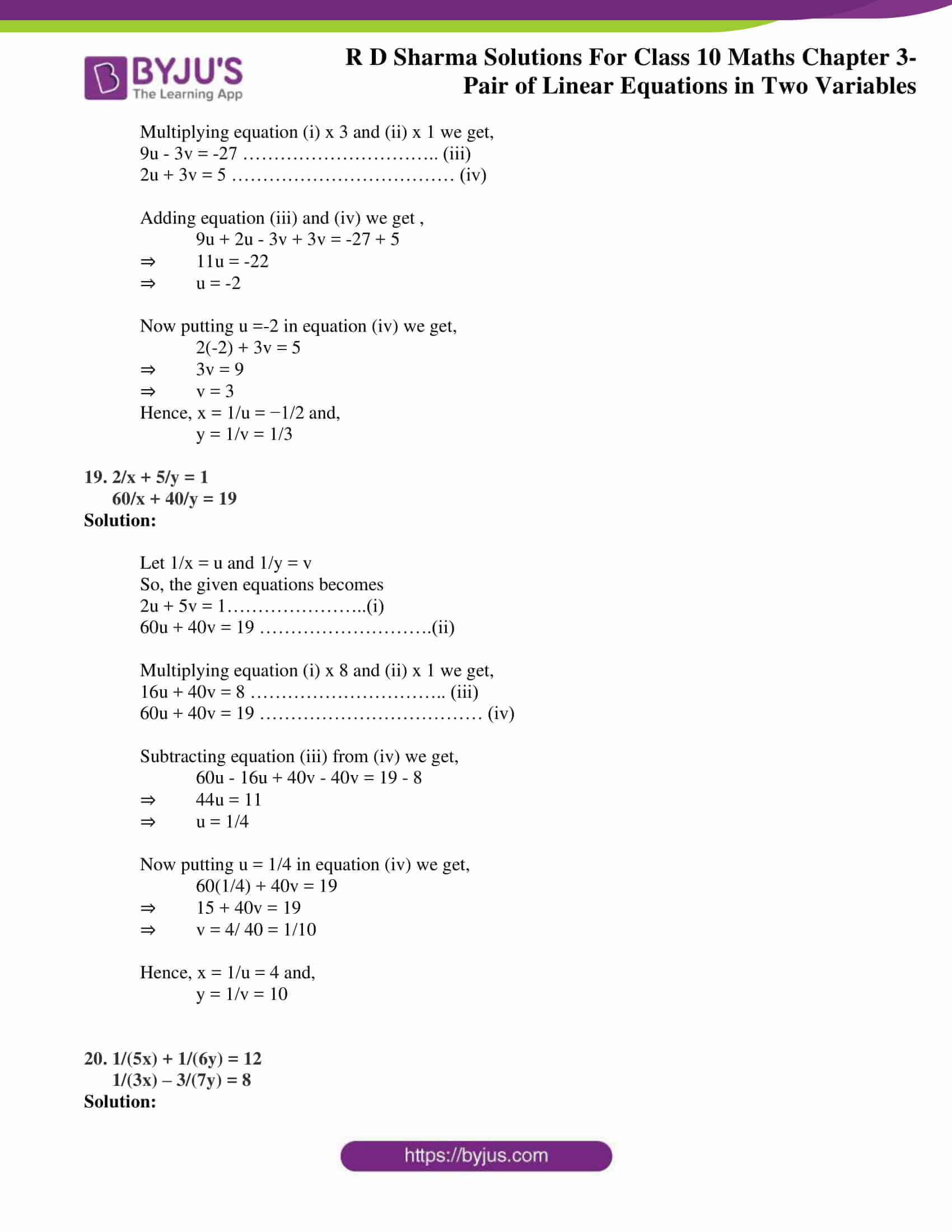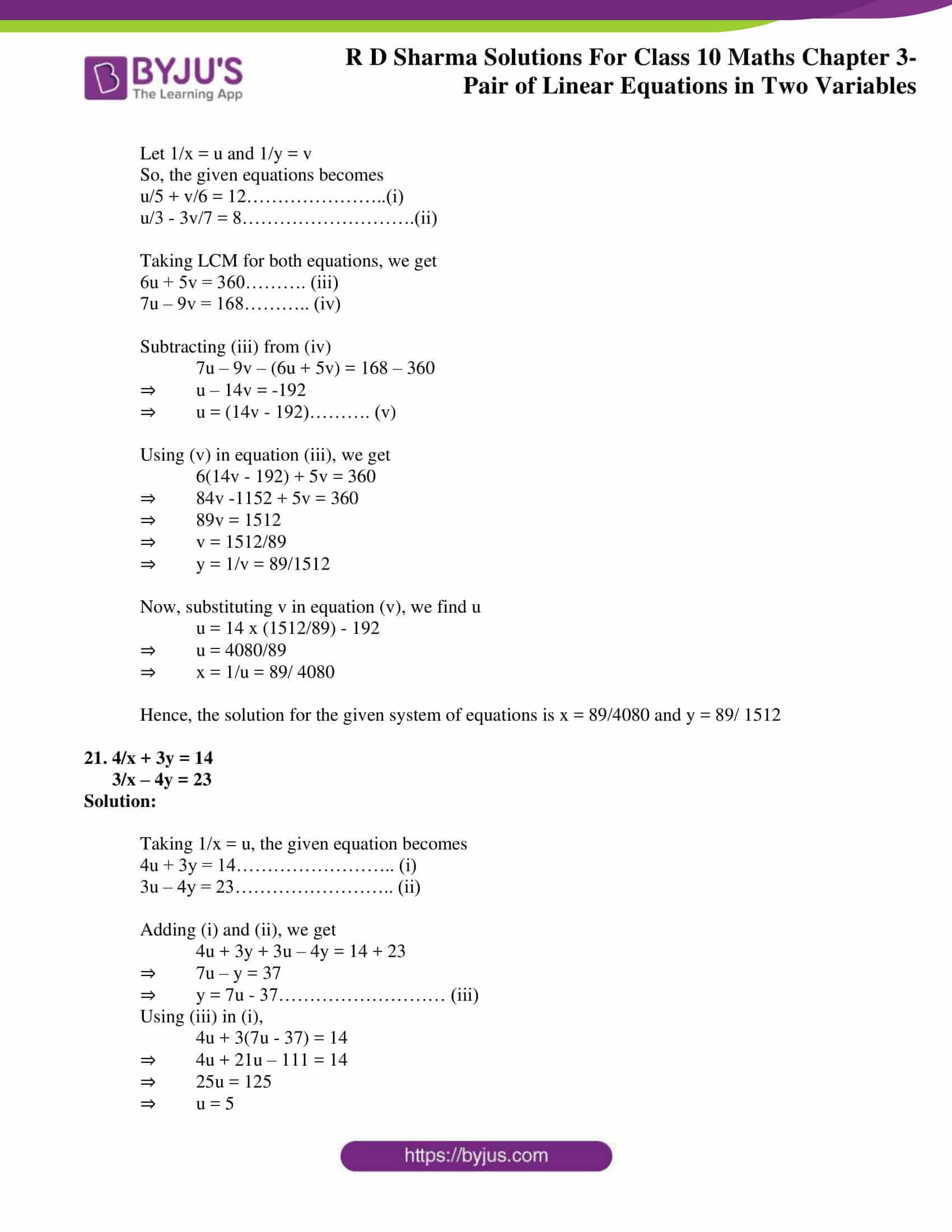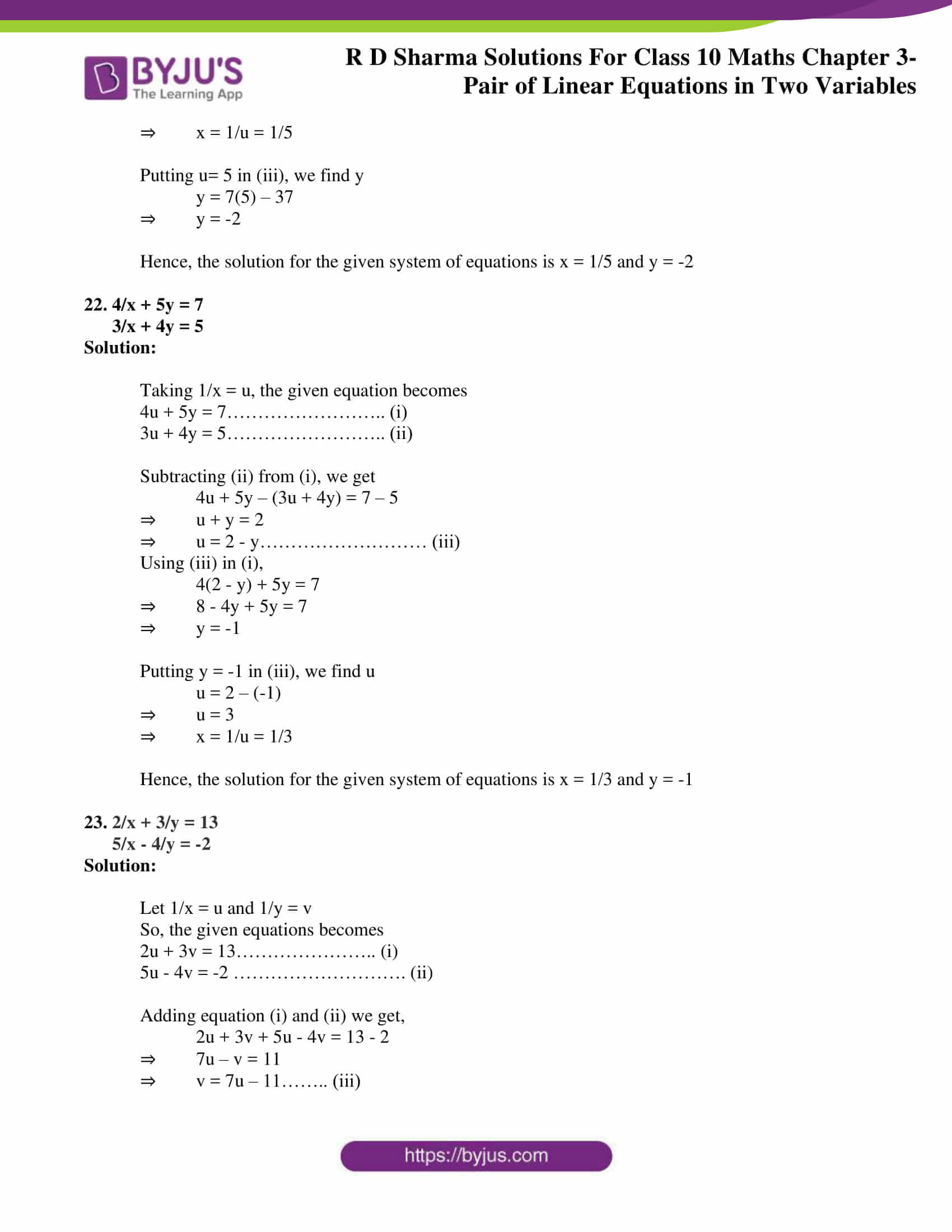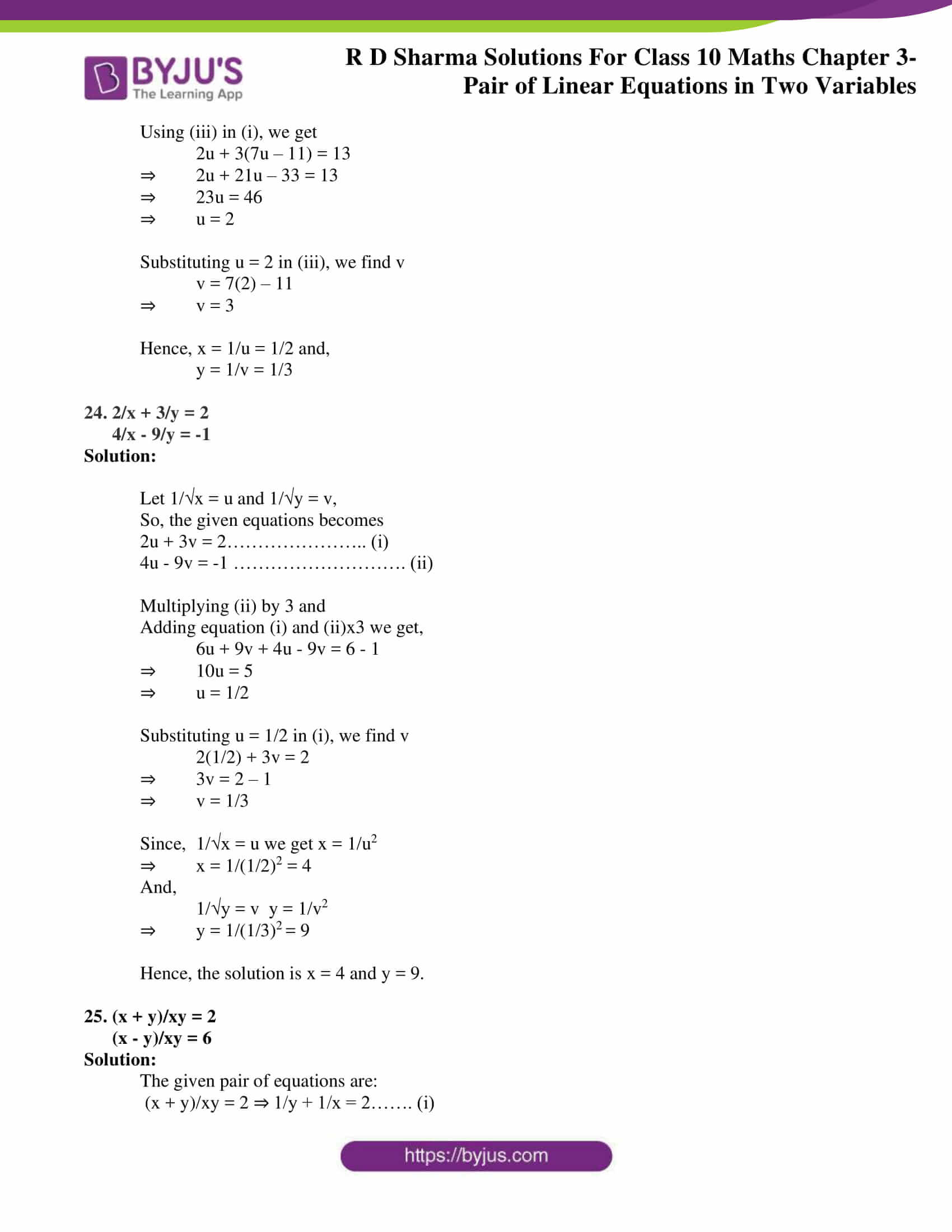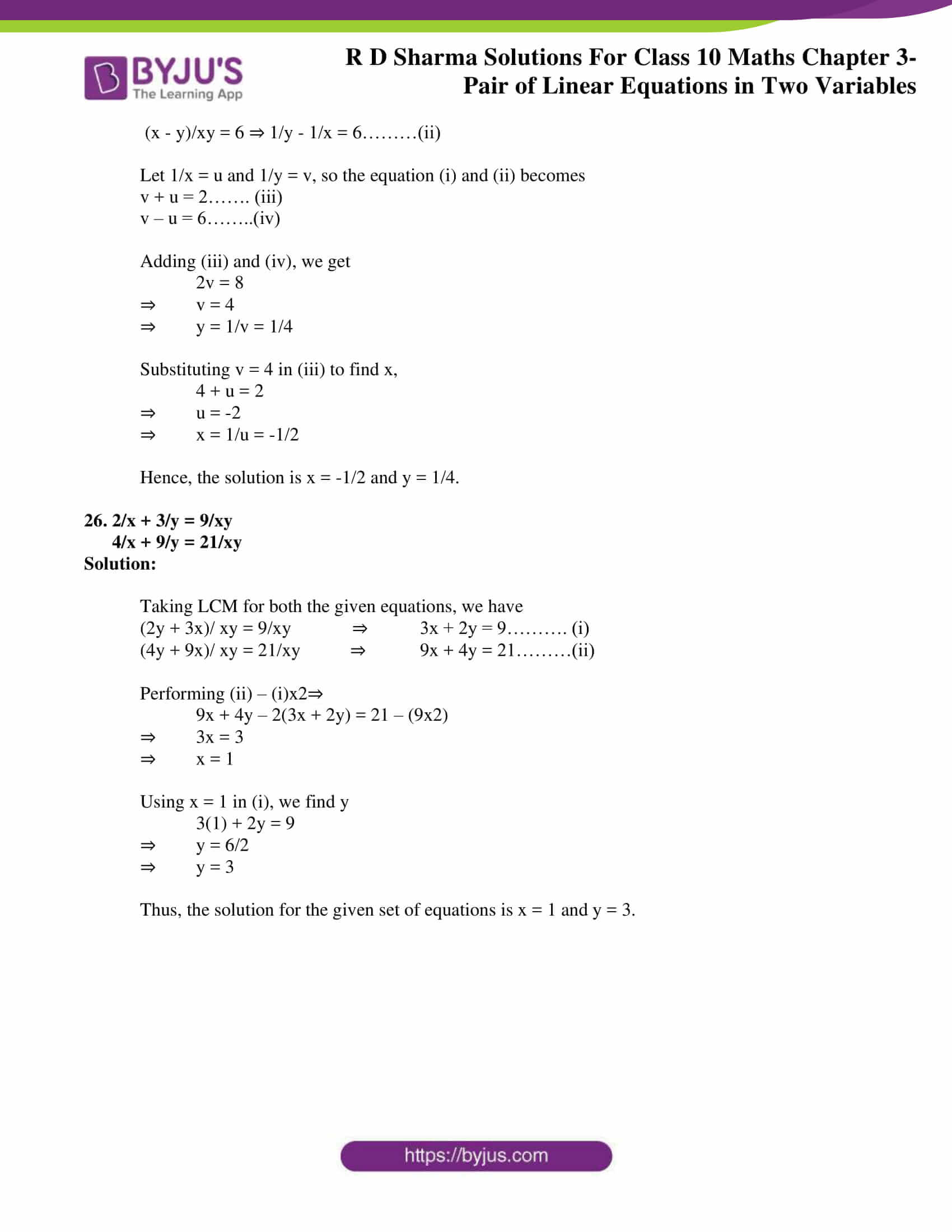### Access RD Sharma Solutions for Class 10 Maths Chapter 3 Pair of Linear Equations In Two Variables Exercise 3.3

Solve the following system of equations:

1. 11x + 15y + 23 = 0

7x – 2y – 20 = 0

Solution:

The given pair of equations are:

11x +15y + 23 = 0 …………………………. (i)

7x – 2y – 20 = 0 …………………………….. (ii)

From (ii)

2y = 7x – 20

⇒ y = (7x −20)/2 ……………………………… (iii)

Now, substituting y in equation (i) we get,

⇒ 11x + 15((7x−20)/2) + 23 = 0

⇒ 11x + (105x − 300)/2 + 23 = 0

⇒ (22x + 105x – 300 + 46) = 0

⇒ 127x – 254 = 0

⇒ x = 2

Next, putting the value of x in the equation (iii) we get,

⇒ y = (7(2) − 20)/2

∴ y= -3

Thus, the value of x and y is found to be 2 and -3 respectively.

2.  3x – 7y + 10 = 0

y – 2x – 3 = 0

Solution:

The given pair of equations are:

3x – 7y + 10 = 0 …………………………. (i)

y – 2x – 3 = 0 ……………………………….. (ii)

From (ii)

y – 2x – 3 = 0

y = 2x+3 ……………………………… (iii)

Now, substituting y in equation (i) we get,

⇒ 3x – 7(2x+3) + 10 = 0

⇒ 3x – 14x – 21 + 10 = 0

⇒ -11x = 11

⇒ x = -1

Next, putting the value of x in the equation (iii) we get,

⇒ y = 2(-1) + 3

∴ y= 1

Thus, the value of x and y is found to be -1 and 1 respectively.

3. 0.4x + 0.3y = 1.7

0.7x – 0.2y = 0.8

Solution:

The given pair of equations are:

0.4x + 0.3y = 1.7

0.7x – 0.2y = 0.8

Let’s, multiply LHS and RHS by 10 to make the coefficients as an integer

4x + 3y = 17 ……………………….. (i)

7x – 2y = 8 …………………………… (ii)

From (ii)

7x – 2y = 8

x = (8 + 2y)/7……………………………… (iii)

Now, substituting x in equation (i) we get,

⇒ 4[(8 + 2y)/7] + 3y = 17

⇒ 32 + 8y + 21y = (17 x 7)

⇒ 29y = 87

⇒ y = 3

Next, putting the value of y in the equation (iii) we get,

⇒ x = (8 + 2(3))/ 7

⇒ x = 14/7

∴ x = 2

Thus, the value of x and y is found to be 2 and 3 respectively.

4. x/2 + y = 0.8

7/(x+ y/2) = 10

Solution:

The given pair of equations are:

x/2 + y = 0.8 ⇒ x + 2y = 1.6…… (a)

7/(x + y/2) = 10 ⇒7 = 10(x + y/2) ⇒7 = 10x + 5y

Let’s, multiply LHS and RHS of equation (a) by 10 for easy calculation

So, we finally get

10x + 20y = 16 ……………………….. (i) And,

10x + 5y = 7 …………………………… (ii)

Now, subtracting two equations we get,

⇒ (i) – (ii)

15y = 9

⇒ y = 3/5

Next, putting the value of y in the equation (i) we get,

x = [16 − 20(3/5)]/10

⇒  (16 – 12)/10 = 4/10

∴ x = 2/5

Thus, the value of x and y obtained are 2/5 and 3/5 respectively.

5. 7(y + 3) – 2(x + 2) = 14

4(y – 2) + 3(x – 3) = 2

Solution:

The given pair of equations are:

7(y+3) – 2(x+2) = 14…………………………. (i)

4(y-2) + 3(x-3) = 2……………………………….. (ii)

From (i), we get

7y + 21 – 2x – 4 = 14

7y = 14 + 4 – 21 + 2x

⇒ y = (2x – 3)/7

From (ii), we get

4y – 8 + 3x – 9 = 2

4y + 3x – 17 – 2 = 0

⇒ 4y + 3x – 19 = 0 …………….. (iii)

Now, substituting y in equation (iii)

4[(2x − 3)/7] + 3x – 19=0

8x – 12 + 21x – (19 x 17) = 0 [after taking LCM]

29x = 145

⇒ x = 5

Now, putting the value of x and in the equation (ii)

4(y-2) + 3(5-3) = 2

⇒ 4y -8 + 6 = 2

⇒ 4y = 4

∴ y = 1

Thus, the value of x and y obtained are 5 and 1 respectively.

6. x/7 + y/3 = 5

x/2 – y/9 = 6

Solution:

The given pair of equations are:

x/7 + y/3 = 5…………………………. (i)

x/2 – y/9 = 6………………………………..(ii)

From (i), we get

x/7 + y/3 = 5

⇒3x + 7y = (5×21) [After taking LCM]

⇒ 3x =105 – 7y

⇒ x = (105 – 7y)/3……. (iv)

From (ii), we get

x/2 – y/9 = 6

⇒ 9x – 2y = 108 ……………………… (iii) [After taking LCM]

Now, substituting x in equation (iii) we get,

9[(105 − 7y)/3] – 2y = 108

⇒ 945 – 63y – 6y = 324 [After taking LCM]

⇒ 945 – 324 = 69y

⇒ 69y = 621

⇒ y = 9

Now, putting the value of y in the equation (iv)

x = (105 − 7(9))/3

⇒ x = (105 − 63)/3 = 42/3

∴ x = 14

Thus, the value of x and y obtained are 14 and 9 respectively.

7. x/3 + y/4 = 11

5x/6 − y/3 = −7

Solution:

The given pair of equations are:

x/3 + y/4 = 11…………………………. (i)

5x/6 − y/3 = −7……………………………….. (ii)

From (i), we get

x/3 + y/4 = 11

⇒4x + 3y = (11×12) [After taking LCM]

⇒ 4x =132 – 3y

⇒ x = (132 – 3y)/4……. (iv)

From (ii), we get

5x/6 − y/3 = −7

⇒ 5x – 2y = -42 ……………………… (iii) [After taking LCM]

Now, substituting x in equation (iii) we get,

5[(132 − 3y)/4] – 2y = -42

⇒ 660 – 15y – 8y = -42 x 4 [After taking LCM]

⇒ 660 + 168 = 23y

⇒ 23y = 828

⇒ y = 36

Now, putting the value of y in the equation (iv)

x = (132 – 3(36))/4

⇒ x = (132 − 108)/4 = 24/4

∴ x = 6

Thus, the value of x and y obtained are 6 and 36 respectively.

8. 4/x + 3y = 8

6/x −4y = −5

Solution:

Taking 1/x = u

Then the two equation becomes,

4u + 3y = 8…………………… (i)

6u – 4y = -5……………………. (ii)

From (i), we get

4u = 8 – 3y

⇒ u = (8 − 3y)/4 …….. (iii)

Substituting u in (ii)

[6(8 − 3y)/4] – 4y = -5

⇒  [3(8−3y)/2] − 4y = −5

⇒ 24 − 9y −8y = −5 x 2 [After taking LCM]

⇒ 24 – 17y = -10

⇒ -17y =- 34

⇒ y = 2

Putting y=2 in (iii) we get,

u = (8 − 3(2))/4

⇒ u = (8 − 6)/4

⇒ u = 2/4 = 1/2

⇒ x = 1/u = 2

∴ x = 2

So, the solution of the pair of equations given is x=2 and y =2.

9. x + y/2 = 4

2y + x/3 = 5

Solution:

The given pair of equations are:

x + y/2 = 4 ……………………. (i)

2y + x/3 = 5……………………. (ii)

From (i) we get,

x + y/2 = 4

⇒ 2x + y = 8 [After taking LCM]

⇒ y = 8 – 2x …..(iv)

From (ii) we get,

x + 6y = 15 ……………… (iii) [After taking LCM]

Substituting y in (iii), we get

x + 6(8 – 2x) = 15

⇒ x + 48 – 12x = 15

⇒ -11x = 15 – 48

⇒ -11x = -33

⇒ x = 3

Putting x = 3 in (iv), we get

y = 8 – (2×3)

∴ y = 8 – 6 = 2

Hence, the solution of the given system of equation are x = 3 and y = 2 respectively.

10. x + 2y = 3/2

2x + y = 3/2

Solution:

The given pair of equations are:

x + 2y = 3/2 …………………. (i)

2x + y = 3/2…………………… (ii)

Let us eliminate y from the given equations. The coefficients of y in the given equations are 2 and 1 respectively. The L.C.M of 2 and 1 is 2. So, we make the coefficient of y equal to 2 in the two equations.

Multiplying equation (i)x1 and (ii)x2 ⇒

x + 2y = 3/2 ………………………. (iii)

4x + 2y = 3 ……………………………………………………. (iv)

Subtracting equation (iii) from (iv)

(4x – x) + (2y-2y) = 3x = 3 – (3/2)

⇒ 3x = 3/2

⇒ x = 1/2

Putting x = 1/2 in equation (iv)

4(1/2) + 2y = 3

⇒ 2 + 2y = 3

∴ y= 1/2

The solution of the system of equation is x = 1/2 and y = 1/2

11. √2x – √3y = 0

√3x − √8y = 0

Solution:

The given pair of equations are:

√2x – √3y = 0……………………….. (i)

√3x − √8y = 0……………………….. (ii)

From equation (i)

x = √(3/2)y ……………..(iii)

Substituting this value in equation (ii) we obtain

√3x − √8y = 0

⇒ √3(√(3/2)y)  − √8y = 0

⇒ (3/√2)y – √8y = 0

⇒ 3y – 4y = 0

⇒ y = 0

Now, substituting y in equation (iii) we obtain

⇒ x=0

Thus, the value of x and y obtained are 0 and 0 respectively.

12. 3x – (y + 7)/11 + 2 = 10

2y + (x + 11)/7 = 10

Solution:

The given pair of equations are:

3x – (y + 7)/11 + 2 = 10……………….. (i)

2y + (x + 11)/7 = 10…………………….. (ii)

From equation (i)

33x – y – 7 + 22 = (10 x 11) [After taking LCM]

⇒ 33x – y + 15 = 110

⇒ 33x + 15 – 110 = y

⇒ y = 33x – 95………. (iv)

From equation (ii)

14 + x + 11 = (10 x 7) [After taking LCM]

⇒ 14y + x + 11 = 70

⇒ 14y + x = 70 – 11

⇒ 14y + x = 59 …………………….. (iii)

Substituting (iv) in (iii) we get,

14 (33x – 95) + x = 59

⇒ 462x – 1330 + x = 59

⇒ 463x = 1389

⇒ x = 3

Putting x = 3 in (iii) we get,

⇒ y = 33(3) – 95

∴ y= 4

The solution for the given pair of equations is x = 3 and y = 4 respectively.

13. 2x – (3/y) = 9

3x + (7/y) = 2 ,y ≠ 0

Solution:

The given pair of equations are:

2x – (3/y) = 9……………………………. (i)

3x + (7/y) = 2…………………………… (ii)

Substituting 1/y = u the above equations becomes,

2x – 3u = 9 ………………………..(iii)

3x + 7u = 2………………………..(iv)

From (iii)

2x = 9 + 3u

⇒ x = (9+3u)/2

Substituting the value of x from above in the equation (iv) we get,

3[(9+3u)/2] + 7u = 2

⇒ 27 + 9u + 14u = (2 x 2)

⇒ 27 + 23u = 4

⇒ 23u = -23

⇒ u = -1

So, y = 1/u = -1

And putting u = -1 in x = (9 + 3u)/2 we get,

⇒ x = [9 + 3(−1)]/2 = 6/2

∴ x = 3

The solution of the pair of equations given are y = 3 and x = -1 respectively.

14. 0.5x + 0.7y = 0.74

0.3x + 0.5y = 0.5

Solution:

The given pair of equations are:

0.5x + 0.7y = 0.74……………………… (i)

0.3x – 0.5y = 0.5 ………………………….. (ii)

Now, let’s multiply LHS and RHS by 100 for both (i) and (ii) for making integral coefficients and constants.

(i) x100 ⇒

50x +70y = 74 ……………………….. (iii)

(ii) x100 ⇒

30x + 50y = 50 …………………………… (iv)

From (iii)

50x = 74 – 70y

x = (74−70y)/ 50 ……………………………… (v)

Now, substituting x in equation (iv) we get,

30[(74−70y)/ 50] + 50y = 50

⇒ 222 – 210y + 250y = 250 [After taking LCM]

⇒ 40y = 28

⇒ y = 0.7

Now, by putting the value of y in the equation (v), we get

⇒ x = [74 − 70(0.7)]/ 50=0

⇒ x =25/ 50 = 1/2

∴ x = 0.5

Thus, the value of x and y so obtained are 0.5 and 0.7 respectively.

15. 1/(7x) + 1/(6y) = 3

1/(2x) – 1/(3y) = 5

Solution:

The given pair of equations are:

1/(7x) + 1/(6y) = 3………………………….. (i)

1/(2x) – 1/(3y) = 5……………………………. (ii)

Multiplying (ii) by 1/2 we get,

1/(4x) – 1/(6y) = 52……………………………. (iii)

Now, solving equations (i) and (iii)

1/(7x) + 1/(6y) = 3………………………….. (i)

1/(4x) – 1/(6y) = 5/2……………………………. (iii)

Adding (i) + (iii) we get,

1/x(1/7 + 1/4 ) = 3 + 5/2

⇒ 1/x(11/28) = 11/2

⇒ x = 1/14

Using x =1/ 14 we get, in (i)

1/[7(1/14)] + 1/(6y) = 3

⇒ 2 + 1/(6y)=3

⇒ 1/(6y) = 1

⇒ y = 1/6

The solution for the given pair of equations is x=1/14 and y=1/6 respectively.

16. 1/(2x) + 1/(3y) = 2

1/(3x) + 1/(2y) = 13/6

Solution:

Let 1/x = u and 1/y = v,

So the given equations becomes,

u/2 + v/3 = 2 ………………(i)

u/3 + v/2 = 13/6 ……………(ii)

From (i), we get

u/2 + v/3 = 2

⇒ 3u + 2v = 12

⇒ u = (12 – 2v)/3 ………….(iii)

Using (iii) in (ii)

[(12 – 2v)/3]/3 + v/2 = 13/6

⇒ (12 – 2v)/9 + v/2 = 13/6

⇒ 24 – 4v + 9v = (13/6) x 18 [after taking LCM]

⇒ 24 + 5v = 39

⇒ 5v = 15

⇒ v = 3

Substituting v in (iii)

u = (12 – 2(3))/3

⇒ u = 2

Thus, x = 1/u ⇒ x = 1/2 and

y = 1/v ⇒ y = 1/3

The solution for the given pair of equations is x = 1/2 and y = 1/3 respectively.

17. 15/u + 2/v = 17

1/u + 1/v = 36/5

Solution:

Let 1/x = u and 1/y = v

So, the given equations becomes

15x + 2y = 17 ………………………….. (i)

x + y = 36/5………………………. (ii)

From equation (i) we get,

2y = 17 – 15x

=y = (17 − 15x)/ 2 …………………. (iii)

Substituting (iii) in equation (ii) we get,

= x + (17 − 15x)/ 2 = 36/5

2x + 17 – 15x = (36 x 2)/ 5 [after taking LCM]

-13x = 72/5 – 17

= -13x = -13/5

⇒ x = 1/5

⇒ u = 1/x = 5

Putting x = 1/5in equation (ii) , we get

1/5 + y = 36/5

⇒ y = 7

⇒ v = 1/y = 1/7

The solution of the pair of equations given are u = 5 and v = 1/7 respectively.

18. 3/x – 1/y = −9

2/x + 3/y = 5

Solution:

Let 1/x = u and 1/y = v

So, the given equations becomes

3u – v = -9…………………..(i)

2u + 3v = 5 ……………………….(ii)

Multiplying equation (i) x 3 and (ii) x 1 we get,

9u – 3v = -27 ………………………….. (iii)

2u + 3v = 5 ……………………………… (iv)

Adding equation (iii) and (iv) we get ,

9u + 2u – 3v + 3v = -27 + 5

⇒ 11u = -22

⇒ u = -2

Now putting u =-2 in equation (iv) we get,

2(-2) + 3v = 5

⇒ 3v = 9

⇒ v = 3

Hence, x = 1/u = −1/2 and,

y = 1/v = 1/3

19. 2/x + 5/y = 1

60/x + 40/y = 19

Solution:

Let 1/x = u and 1/y = v

So, the given equations becomes

2u + 5v = 1…………………..(i)

60u + 40v = 19 ……………………….(ii)

Multiplying equation (i) x 8 and (ii) x 1 we get,

16u + 40v = 8 ………………………….. (iii)

60u + 40v = 19 ……………………………… (iv)

Subtracting equation (iii) from (iv) we get,

60u – 16u + 40v – 40v = 19 – 8

⇒ 44u = 11

⇒ u = 1/4

Now putting u = 1/4 in equation (iv) we get,

60(1/4) + 40v = 19

⇒ 15 + 40v = 19

⇒ v = 4/ 40 = 1/10

Hence, x = 1/u = 4 and,

y = 1/v = 10

20. 1/(5x) + 1/(6y) = 12

1/(3x) – 3/(7y) = 8

Solution:

Let 1/x = u and 1/y = v

So, the given equations becomes

u/5 + v/6 = 12…………………..(i)

u/3 – 3v/7 = 8……………………….(ii)

Taking LCM for both equations, we get

6u + 5v = 360………. (iii)

7u – 9v = 168……….. (iv)

Subtracting (iii) from (iv)

7u – 9v – (6u + 5v) = 168 – 360

⇒ u – 14v = -192

⇒ u = (14v – 192)………. (v)

Using (v) in equation (iii), we get

6(14v – 192) + 5v = 360

⇒ 84v -1152 + 5v = 360

⇒ 89v = 1512

⇒ v = 1512/89

⇒ y = 1/v = 89/1512

Now, substituting v in equation (v), we find u

u = 14 x (1512/89) – 192

⇒ u = 4080/89

⇒ x = 1/u = 89/ 4080

Hence, the solution for the given system of equations is x = 89/4080 and y = 89/ 1512

21. 4/x + 3y = 14

3/x – 4y = 23

Solution:

Taking 1/x = u, the given equation becomes

4u + 3y = 14…………………….. (i)

3u – 4y = 23…………………….. (ii)

Adding (i) and (ii), we get

4u + 3y + 3u – 4y = 14 + 23

⇒ 7u – y = 37

⇒ y = 7u – 37……………………… (iii)

Using (iii) in (i),

4u + 3(7u – 37) = 14

⇒ 4u + 21u – 111 = 14

⇒ 25u = 125

⇒ u = 5

⇒ x = 1/u = 1/5

Putting u= 5 in (iii), we find y

y = 7(5) – 37

⇒ y = -2

Hence, the solution for the given system of equations is x = 1/5 and y = -2

22. 4/x + 5y = 7

3/x + 4y = 5

Solution:

Taking 1/x = u, the given equation becomes

4u + 5y = 7…………………….. (i)

3u + 4y = 5…………………….. (ii)

Subtracting (ii) from (i), we get

4u + 5y – (3u + 4y) = 7 – 5

⇒ u + y = 2

⇒ u = 2 – y……………………… (iii)

Using (iii) in (i),

4(2 – y) + 5y = 7

⇒ 8 – 4y + 5y = 7

⇒ y = -1

Putting y = -1 in (iii), we find u

u = 2 – (-1)

⇒ u = 3

⇒ x = 1/u = 1/3

Hence, the solution for the given system of equations is x = 1/3 and y = -1

23. 2/x + 3/y = 13

5/x – 4/y = -2

Solution:

Let 1/x = u and 1/y = v

So, the given equations becomes

2u + 3v = 13………………….. (i)

5u – 4v = -2 ………………………. (ii)

Adding equation (i) and (ii) we get,

2u + 3v + 5u – 4v = 13 – 2

⇒ 7u – v = 11

⇒ v = 7u – 11…….. (iii)

Using (iii) in (i), we get

2u + 3(7u – 11) = 13

⇒ 2u + 21u – 33 = 13

⇒ 23u = 46

⇒ u = 2

Substituting u = 2 in (iii), we find v

v = 7(2) – 11

⇒ v = 3

Hence, x = 1/u = 1/2 and,

y = 1/v = 1/3

24. 2/x + 3/y = 2

4/x – 9/y = -1

Solution:

Let 1/√x = u and 1/√y = v,

So, the given equations becomes

2u + 3v = 2………………….. (i)

4u – 9v = -1 ………………………. (ii)

Multiplying (ii) by 3 and

Adding equation (i) and (ii)x3 we get,

6u + 9v + 4u – 9v = 6 – 1

⇒ 10u = 5

⇒ u = 1/2

Substituting u = 1/2 in (i), we find v

2(1/2) + 3v = 2

⇒ 3v = 2 – 1

⇒ v = 1/3

Since, 1/√x = u we get x = 1/u2

⇒ x = 1/(1/2)2 = 4

And,

1/√y = v y = 1/v2

⇒ y = 1/(1/3)2 = 9

Hence, the solution is x = 4 and y = 9.

25. (x + y)/xy = 2

(x – y)/xy = 6

Solution:

The given pair of equations are:

(x + y)/xy = 2 ⇒ 1/y + 1/x = 2……. (i)

(x – y)/xy = 6 ⇒ 1/y – 1/x = 6………(ii)

Let 1/x = u and 1/y = v, so the equation (i) and (ii) becomes

v + u = 2……. (iii)

v – u = 6……..(iv)

Adding (iii) and (iv), we get

2v = 8

⇒ v = 4

⇒ y = 1/v = 1/4

Substituting v = 4 in (iii) to find x,

4 + u = 2

⇒ u = -2

⇒ x = 1/u = -1/2

Hence, the solution is x = -1/2 and y = 1/4.

26. 2/x + 3/y = 9/xy

4/x + 9/y = 21/xy

Solution:

Taking LCM for both the given equations, we have

(2y + 3x)/ xy = 9/xy ⇒ 3x + 2y = 9………. (i)

(4y + 9x)/ xy = 21/xy ⇒ 9x + 4y = 21………(ii)

Performing (ii) – (i)x2⇒

9x + 4y – 2(3x + 2y) = 21 – (9×2)

⇒ 3x = 3

⇒ x = 1

Using x = 1 in (i), we find y

3(1) + 2y = 9

⇒ y = 6/2

⇒ y = 3

Thus, the solution for the given set of equations is x = 1 and y = 3.